# Flow through an explicitly fractured medium and flow through a fractured network

Philipp Sch&#228;dle, Andy Wilkins, Anozie Ebigbo and Martin O Saar

Example files are in flow_through_fractured_media.

## Introduction

The PorousFlow module may be used to simulate flow (both fluid and heat) through a medium that contains explicit fractures. This may be performed in two ways:

1. A simulation may use a 3D mesh that contains thin 3D elements to represent the fractures. Usually the thin "fracture" elements are part of a separate block in the mesh to which appropriate porosity and permeability are prescribed. No special input-file magic needs to be performed. The same remarks hold for a 2D mesh containing thin 2D elements representing the fractures.

2. A simulation may use a 3D mesh that contains 2D elements to represent the fractures. Of course this is slightly more numerically efficient than the aforementioned method, but, most importantly, the mesh is usually easier to create. This example concentrates on this method. Similar remarks hold for a 2D mesh containing 1D elements. This method is only valid if flow across the fracture is very quick compared with flow through the bulk. For instance, the method is valid for highly conductive fractures, but is not suitable for modelling aquitards. This is because the 2D fracture does not prevent fluid from moving across it: fluid/heat moving in the direction normal to the fracture does not even see the fracure.

An overview of the mixed-dimensional method is presented along with a verification of the results for solute transport along a single fracture embedded in a porous matrix. Some important remarks concerning the retardation effect of the porous matrix are also made below.

An identical method may be used to simulate flow through a fractured network (ie, 2D elements without the "bulk" 3D elements).

## The mesh

The key aspect of the formulation presented here is the mesh. It is a mixed-dimensional, conforming mesh. That is, the mesh needs to be generated such that the lower-dimensional fracture elements share nodes with the porous-matrix elements. On these nodes, computational variables take on a single value, and the nodes physically connect the subdomains. In real fractured materials a good mesh may be very difficult to create because of the complicated intersection of many different fractures.

Figure 1 shows an example of the required configuration for the conforming mesh and the different sub-domains.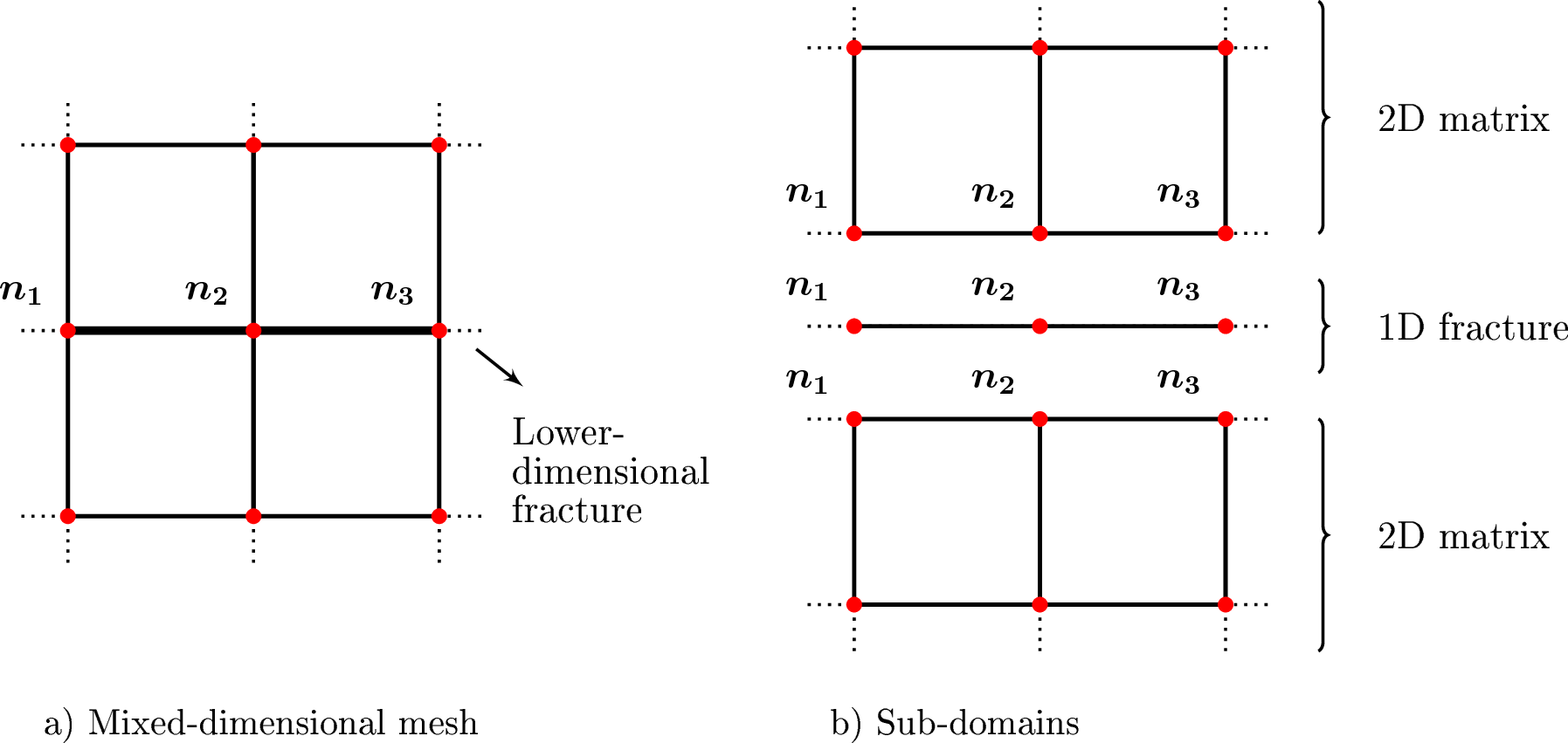Figure 1: (a): The mesh used by MOOSE, which is a mixed-dimensional conforming mesh containing 1D and 2D elements. (b): An exploded view. Nodes , , need to be shared by the three domains.

When creating a mesh:

• For the case of 2D elements embedded in a 3D porous matrix, the 2D elements need to be QUAD or TRISHELL3 elements that are connected to the 3D elements. (example journal file: coarse_3D.jou)

• For the case of 1D elements embedded in a 2D porous matrix, the 1D elements need to be BAR elements that are connected to 2D QUAD or TRI3 elements. (example journal file: coarse.jou)

• In the case of an isolated fracture network, the mesh needs to consist of QUAD or TRI3 elements (for 2D fractures) or BAR elements (for 1D fractures).

When creating a 3D mesh with 2D fracture elements of type TRISHELL3 in Trelis/Cubit:

• During meshing Trelis/Cubit might change the element type from TRISHELL3 to TRI3. However, Trelis/Cubit keeps the correct element sides that are generated for the TRISHELL3 elements. This is certainly due to a bug in some Trelis/Cubit versions and is not a big deal as long as the user is aware of it.

• The element type in produced exodus mesh file needs to be modified manually. The shell script modif_trishell3_exodus_file.sh in the example folder might be used to to this.

Finally, the fractures elements need to be part of a separate subdomain (block). This is actually a requirement of the exodus format, but most importantly it allows permeability, porosity, heat conductivity, etc, to be correctly prescribed.

In this example, the model consists of a 2D domain with a single, 1D fracture embedded in a porous matrix.

## Material properties

The second aspect of the mixed-dimensional method is prescribing appropriate material properties (permeability, porosity, conductivity, etc) to the fracture blocks. Although the details below may seem daunting the conclusion is simple: just multiply the experimentally measured fractured permeability, porosity, etc by the fracture aperture and enter this number in your input file.

### Residuals

Consider the MOOSE residual for a single 3D element corresponding to the time derivative of the fluid mass. It is (1) In this equation is the residual, with the "3" subscript indicating that this is a residual coming from a 3D element, and is the volume of the element. The other notation is detailed in nomenclature.

Similarly, consider the MOOSE residual for a single 2D element corresponding to the time derivative of the fluid mass. It is (2) Here has been used to emphasise that this "2D" porosity is not necessarily the same as the 3D porosity, .

When constructing the overall nonlinear residual, MOOSE sums all the residuals from the individual elements (ie, MOOSE sums all the 's and all the 's). The "2D" porosity must be chosen correctly so that the contributions from are weighted appropriately.

To do this, think of the 2D elements as very thin 3D elements. Assuming there is no dependency on the third direction, as is appropriate because the 3D element is very thin, the residual for these thin elements is (3) where is the thickness in the third direction. We want this to be identical to , which immediately implies (4)

Similar arguments may be made for the fluid flow or heat flow Kernels of PorousFlow (and indeed, the entire PDEs) and these result in equations such as (5) where is the permeability tensor.

The tildered parameter values are those that should be used in the MOOSE input file, for the Material properties of the blocks associated to the 2D elements.

### Example

For instance, suppose that a sample of the fracture is experimentally measured to have porosity . Perhaps this fracture is a sand-filled planar inclusion in a granite rock mass, for instance. Obviously this is a 3D result since it exists in real life. Suppose the fracture is known to have thickness 0.1m. Then when this fracture is represented as a 2D element in MOOSE it should be given porosity (with units of metres).

### Relationship to the var element = document.getElementById("moose-equation-2394d452-ffbe-4682-afc0-a53cabb537e2");katex.render("a^2", element, {displayMode:false,throwOnError:false}); fracture formula - the cubic law

It is known that fluid flow between a pair of impermeable parallel plates may be well-approximated by Darcy flow through an effective medium with permeability , where is the separation of the plates. In this setup the fluid flow is unimpeded by obstructions or similar things between the parallel plates. The parallel plates are not included in this effective medium: it is simply a slab of material with permeability . This effective medium may be represented by 3D elements in MOOSE and simulated in the usual way, by setting the permeability in the input file to .

How is this related to the formulae presented above? Well, the user may set , which would be appropriate if their fracture was well approximated by parallel plates, and then, assuming the fratures are modelled by 2D elements in a 3D mesh, use the formula to prescribe the permeability to the 2D fractures (probably along with which implies ). But users don't strictly _need_ to do this: any that is deemed appropriate may be used, such as a value derived from experimental data measured in real (3D) experiments performed on the material the fracture is made from. Such a value is unlikely to depend on , because it will be dictated by the material present within the fracture (eg, sand) which is assumed not to be present when deriving the formula.

## Verification

### Meshes used

For the verification of this approach, two different simulations are run on two different meshes. The bulk porous material is 2D.

1. In the first simulation, the fracture is geometrically represented by 2D elements with a thickness that corresponds to the aperture of the fracture. This is the reference case with which the lower-dimensional fracture case is to be compared.

2. In the second simulation, the fracture is modeled by lower-dimensional (1D) elements.

The discretization along the fracture is similar in both cases. Further, the discretization in the porous matrix perpendicular to the fracture increases logarithmically and is similar for both models. Figure 2 shows a close-up of the region near the fracture for the two different meshes. The results of these two simulations are compared for verification.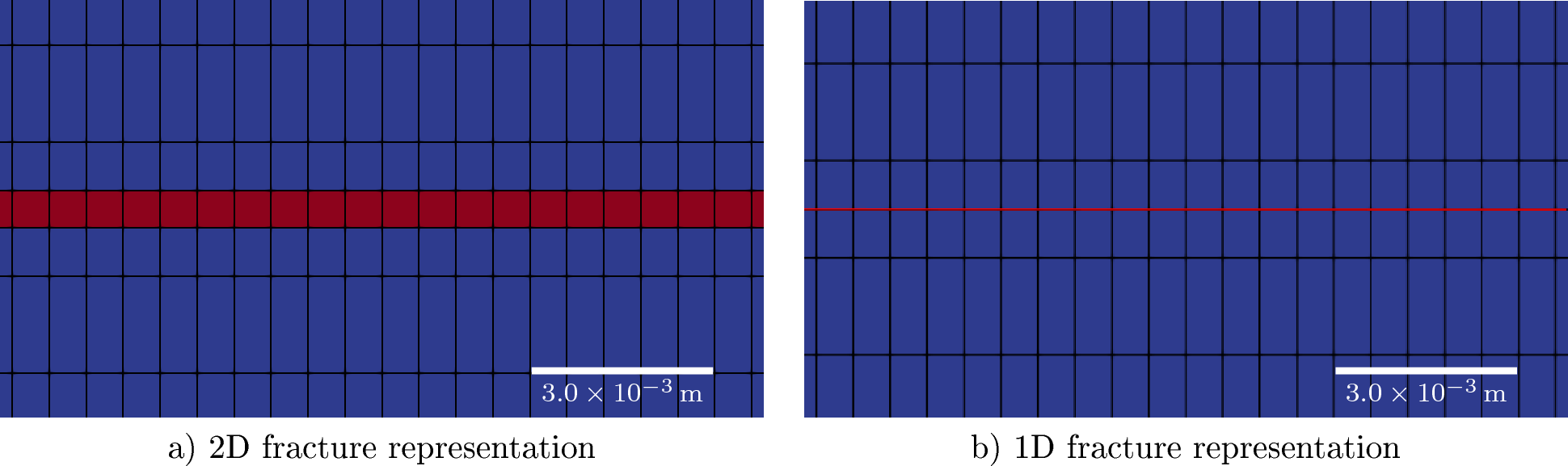Figure 2: Close-up of the area near the fracture (red) in a porous matrix (blue) as represented by the two meshes. In (a), the fracture is represented by 2D elements whose heights correspond to the aperture of the fracture. In (b), the fracture is represented by 1D elements that are embedded in the "bulk" 2D elements.

### Problem description

Single-phase fluid flow and transport of one solute though a fracture and adjacent porous matrix is simulated. The porous matrix is assumed to have a low permeability, so that transport in the matrix is mainly diffusive.

Steady-state flow equations are solved to obtain the pressure field. This is followed by a transient simulation of the transport of the solute. Dirichlet boundary conditions are applied for pressure and solute mass fraction at the left and right ends of the fracture. The pressure difference of 0.002MPa results in laminar fluid flow from right to left. Similarly, the mass fraction at the right boundary is and at the left boundary . The dimensions of the model are 1m along the fracture and 0.2m perpendicular to the fracture.

For the 1D fracture case, it is assumed that the fracture aperture is constant within each fracture element. Hence, in order to account for the missing dimension, the 1D mass balance equation is multiplied by the aperture. This is realized in the MOOSE input file by multiplying the parameters for fracture porosity and fracture permeability by the aperture as described Material properties.

The permeability of the fracture is calculated as a function of aperture, , using the cubic law: (6) The remaining relevant input parameters are tabulated in Table 1

Table 1: Input parameters

ParameterCase with 2D fracturesCase with 1D fractures
Aperturemm
Permeability, fracturemm
Permeability, matrixmm
Porosity, fracturem
Porosity, matrix
Tortuosity, fracture11
Tortuosity, matrix0.10.1
Diffusion coefficientm.sm.s

The important parts of the mixed-dimensional input file are

  [./permeability_fracture]
type = PorousFlowPermeabilityConst
permeability = '1.8e-11 0 0 0 1.8e-11 0 0 0 1.8e-11' # kf=3e-8, a=6e-4m.  1.8e-11 = kf * a
block = 'fracture'
[../]
[./permeability_matrix]
type = PorousFlowPermeabilityConst
permeability = '1e-20 0 0 0 1e-20 0 0 0 1e-20'
block = 'matrix1 matrix2'
[../]

(modules/porous_flow/examples/flow_through_fractured_media/fine_transient.i)
  [./poro_fracture]
type = PorousFlowPorosityConst
porosity = 6e-4   # = a * phif
block = 'fracture'
[../]
[./poro_matrix]
type = PorousFlowPorosityConst
porosity = 0.1
block = 'matrix1 matrix2'
[../]
[./diff1]
type = PorousFlowDiffusivityConst
diffusion_coeff = '1e-9 1e-9'
tortuosity = 1.0
block = 'fracture'
[../]
[./diff2]
type = PorousFlowDiffusivityConst
diffusion_coeff = '1e-9 1e-9'
tortuosity = 0.1
block = 'matrix1 matrix2'
[../]
[./relp]
type = PorousFlowRelativePermeabilityConst
phase = 0
[../]
[./permeability_fracture]
type = PorousFlowPermeabilityConst
permeability = '1.8e-11 0 0 0 1.8e-11 0 0 0 1.8e-11' # kf=3e-8, a=6e-4m.  1.8e-11 = kf * a
block = 'fracture'
[../]
[./permeability_matrix]
type = PorousFlowPermeabilityConst
permeability = '1e-20 0 0 0 1e-20 0 0 0 1e-20'
block = 'matrix1 matrix2'
[../]
[]

[Functions]
[./dt_controller]
type = PiecewiseConstant
x = '0    30   40 100 200 83200'
y = '0.01 0.1  1  10  100 32'
[../]
[]

[Preconditioning]
active = basic
[./mumps_is_best_for_parallel_jobs]
type = SMP
full = true
petsc_options_iname = '-pc_type -pc_factor_mat_solver_package'
petsc_options_value = ' lu       mumps'
[../]
[./basic]
type = SMP
full = true
petsc_options_iname = '-ksp_type -pc_type -sub_pc_type -sub_pc_factor_shift_type -pc_asm_overlap'
petsc_options_value = 'gmres      asm      lu           NONZERO                   2             '
[../]
[]

[Executioner]
type = Transient
solve_type = NEWTON
end_time = 86400

[./TimeStepper]
type = FunctionDT
function = dt_controller
[../]

# controls for nonlinear iterations
nl_max_its = 15
nl_rel_tol = 1e-14
nl_abs_tol = 1e-9
[]

[VectorPostprocessors]
[./xmass]
type = LineValueSampler
start_point = '0.4 0 0'
end_point = '0.5 0 0'
sort_by = x
num_points = 167
variable = massfrac0
[../]
[]

[Outputs]
perf_graph = true
console = true
csv = true
exodus = true
[]

(modules/porous_flow/examples/flow_through_fractured_media/fine_transient.i)

### AuxKernels

Given the alterations to the input parameters to account for the lower-dimensional domain, it is important to take care when calculating velocities using PorousFlow Auxkernels. The PorousFlowDarcyVelocityComponentLowerDimensional AuxKernel uses the correct gravitational vectors in the lower-dimensional domain. However, the velocities calculated by this AuxKernel only have units of m.s if the aperture is provided to the AuxKernel. The aperture does not need to be supplied to the bulk Darcy velocities computed by PorousFlowDarcyVelocityComponent.

Also note that these are not the velocities seen by an observer watching a tracer move through the medium. To obtain those velocities, the bulk (3D) Darcy velocity must be divided by the porosity. The fracture Darcy velocity must be divided by , or if the aperture is not provided to PorousFlowDarcyVelocityComponentLowerDimensional.

Finally, it is important to note that the velocity AuxVariables defined for the fracture domain should be family=MONOMIAL and order=CONSTANT in order to display accurate velocities using Paraview.

The following mixed-dimensional input-file blocks define Darcy velocity on a lower-dimensional fracture, with the result having units of m.s.

[AuxVariables]
[./velocity_x]
family = MONOMIAL
order = CONSTANT
block = fracture
[../]
[./velocity_y]
family = MONOMIAL
order = CONSTANT
block = fracture
[../]
[]

[AuxKernels]
[./velocity_x]
type = PorousFlowDarcyVelocityComponentLowerDimensional
variable = velocity_x
component = x
aperture = 6E-4
[../]
[./velocity_y]
type = PorousFlowDarcyVelocityComponentLowerDimensional
variable = velocity_y
component = y
aperture = 6E-4
[../]
[]

(modules/porous_flow/examples/flow_through_fractured_media/fine_transient.i)

### Results

Figure 3 and Figure 4 show results of the solute-transport problem. The advection of the solute is shown as well as its penetration into the porous matrix. The results are identical for the two different meshes, demonstrating the correctness of the mixed-dimensional approach.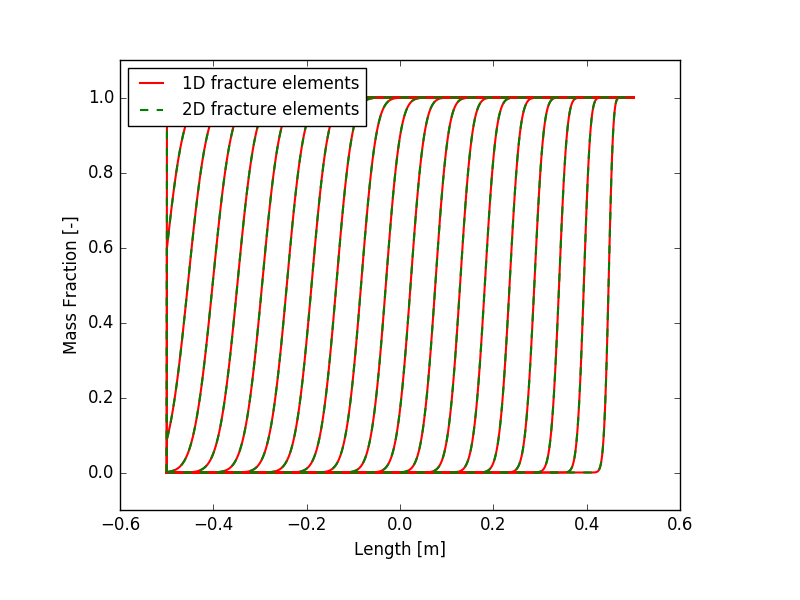Figure 3: Mass fraction along the fracture for each of the first 20 seconds of simulation.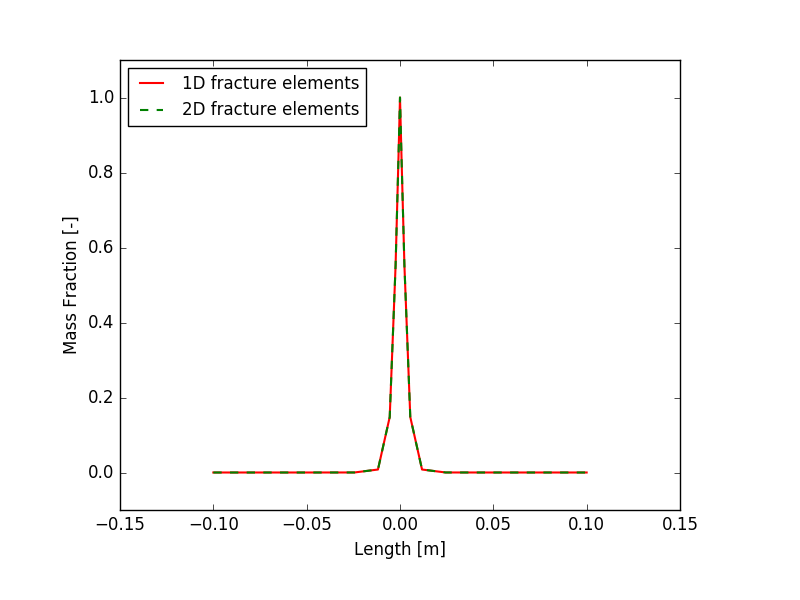Figure 4: Mass fraction perpendicular to the fracture at location 0.1m from the injection point after 24 hours of simulation.

## A 3D example

Identical methods may be used to simulate flow in 3D. An example mesh and an input file may be found in flow_through_fractured_media. Two intersecting eliptical fractures are embedded in a 3D porous material. A porepressure gradient is established, and a tracer is injected at the edge of one of the fractures. The tracer flows mainly along the fractures, but diffuses a little into the bulk material. Figure 5 shows the result at one time, and Figure 6 shows an animation of the tracer concentration.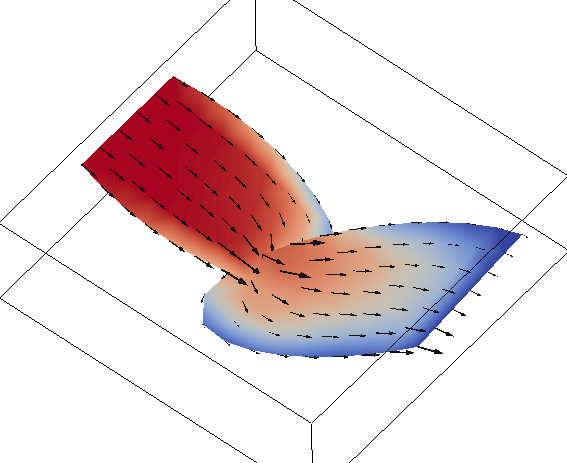Figure 5: Tracer mass fraction (red means high) in two intersecting 2D fractures contained in a 3D porous medium.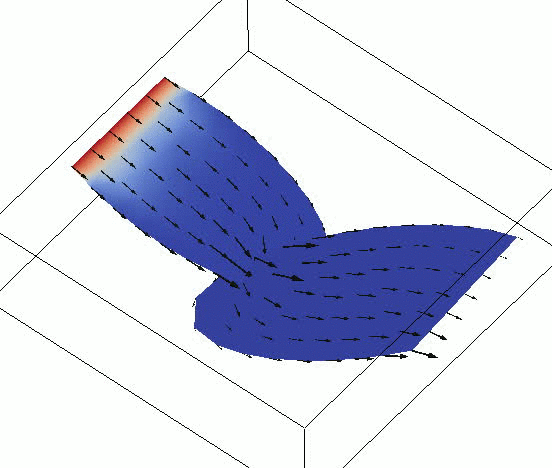Figure 6: Tracer mass fraction in the 2D fractures as time progresses.

## Retardation of the flow by the matrix

The above results demonstrate that a thin 3D fracture may be represented accurately by a 2D fracture, providing the fracture's porosity and permeability (etc) are scaled by the aperture. So, the results are the same for both methods, but are the results actually accurate?

In this section we demonstrate that the neighbouring porous matrix (the 3D blocks) have a "retardation" effect on the fluid/heat flow. This effect is not limited to PorousFlow, or even MOOSE: it is simply a consequence of the way that finite-element solvers work.

Instead of using PorousFlow, consider the simpler heat equation: (7) where is the diffusivity, which is assumed to be constant, but different for the matrix and the fracture. The model that we will study is a 2D model containing a single fracture. Three meshes are used, as shown in Figure 7.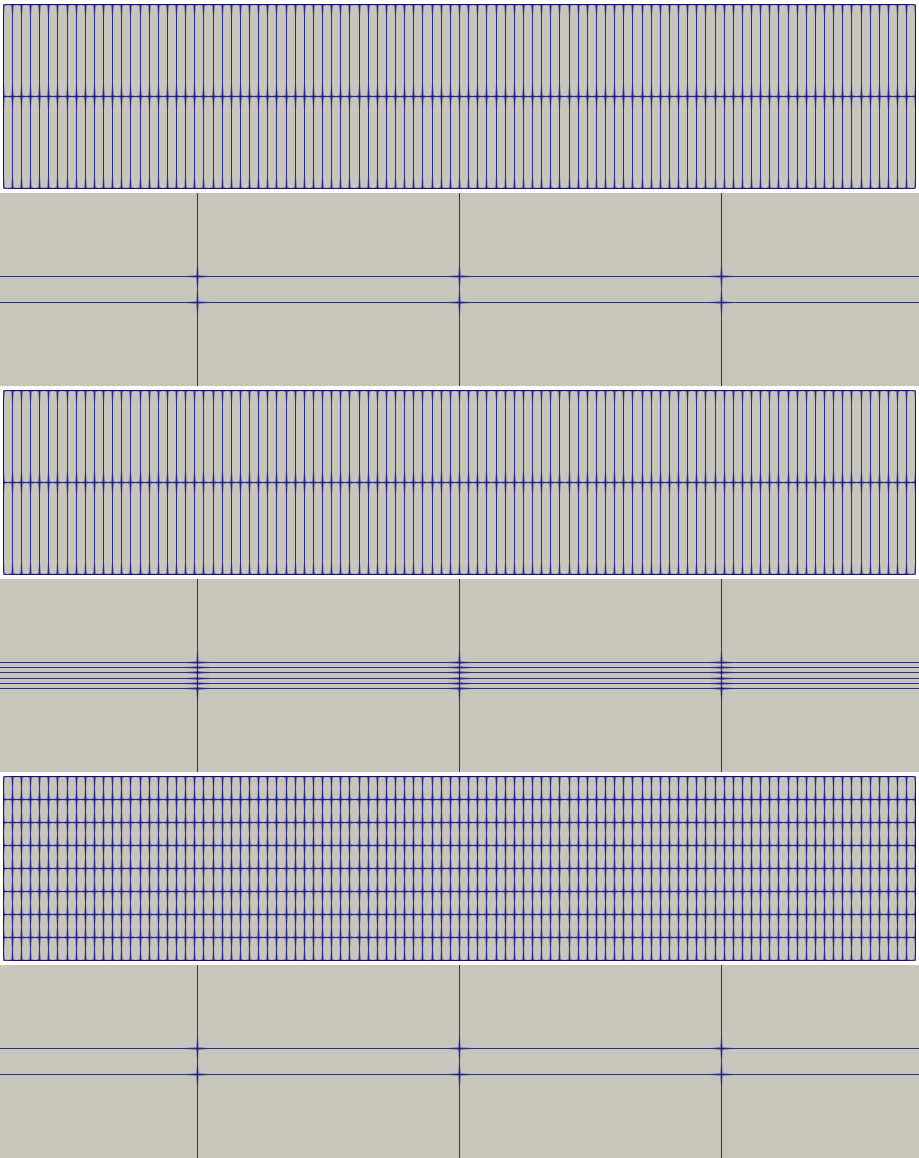Figure 7: The 2D meshes used in the diffusion simulation. Each mesh consists of a central thin 2D fracture surrounded by a large and coarsely-meshed matrix. Top: Mesh A: fracture width meshed with 1 element; matrix with 1 element. Second: Mesh A zoom. Third: Mesh B: fracture width meshed with 5 elements; matrix with 1 element. Fourth: Mesh B zoom. Fifth: Mesh C: fracture width meshed with 1 element; matrix with 4 elements. Bottom: Mesh C zoom.

The temperature is initialized to zero, and fixed to 1 on the left side of the fracture. The fracture has diffusivity 1, while the matrix has diffusivity zero. This means that heat will flow only in the fracture, towards the right-hand-side of the model. The same input file is used for each mesh:

[Mesh]
file = diffusion_1.e # or diffusion_5.e or diffusion_fine.e
[]

[Variables]
[./T]
[../]
[]

[BCs]
[./left]
type = PresetBC
boundary = 2
variable = T
value = 1
[../]
[]

[Kernels]
[./dot]
type = TimeDerivative
variable = T
[../]
[./fracture_diffusion]
type = AnisotropicDiffusion
block = 1
tensor_coeff = '1 0 0  0 1 0  0 0 1'
variable = T
[../]
[./matrix_diffusion]
type = AnisotropicDiffusion
block = '2 3'
tensor_coeff = '0 0 0  0 0 0  0 0 0'
variable = T
[../]
[]

[Preconditioning]
[./entire_jacobian]
type = SMP
full = true
[../]
[]

[Executioner]
type = Transient
solve_type = NEWTON
dt = 10
end_time = 100
[]

[Outputs]
print_linear_residuals = false
exodus = true
[]

(modules/porous_flow/examples/flow_through_fractured_media/diffusion.i)

The results after some time of simulation are shown in Figure 8. Evidently Mesh A and Mesh B give the same result: the discretisation of the fracture makes no difference. However, the temperature diffuses much faster through the fracture when using Mesh C.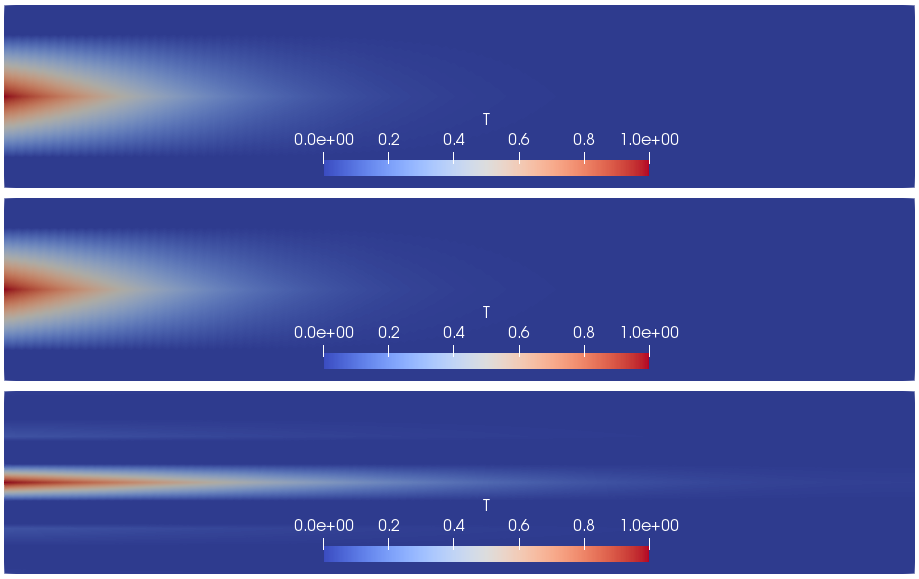Figure 8: Result of diffusion experiment using the 3 different meshes. Top: using Mesh A. Second: using Mesh B. Bottom: using Mesh C.

The reason for this is the following. Heat flows into the system through the fracture's left side. In each mesh it has to "fill up" the nodes on the top and bottom of the fracture. The nodal volume of these nodes contains contributions from the matrix elements that they are joined to. So the nodal volume of the nodes in Mesh A and B is quite large compared to Mesh C. Hence, more heat energy is needed to raise their temperature, which means that the temperature changes more slowly in Mesh A and B compared with Mesh C.

We call this "retardation" of the flow by the matrix. It is not a real physical effect: it is due to the coarse resolution in the matrix. Although this section has concentrated on the simple diffusion equation with no numerical stabilization, exactly the same phenomenom occurs in PorousFlow simulations, and users may need to be aware of this.